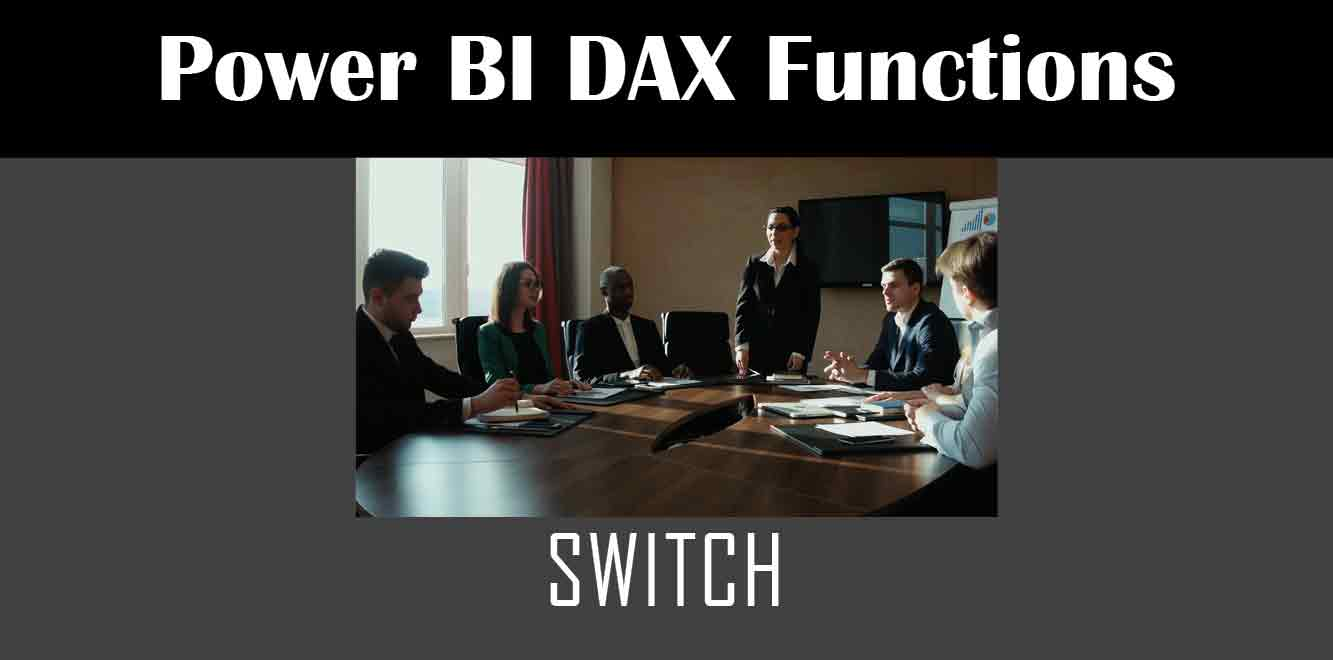# SWITCH

## Y## What is the SWITCH Function in Power BI DAX?

The SWITCH function in Power BI DAX is a conditional expression that takes a specified value and compares it with a series of conditions. If the value matches a condition, the corresponding result is returned. If no condition is met, the default value is returned.

## Syntax of the SWITCH Function

The syntax of the SWITCH function in Power BI DAX is as follows:

SWITCH(Expression,Value1,Result1,Value2,Result2,…,ValueN,ResultN,[DefaultResult])

``` - Expression: This is the value to be evaluated. - Value1, Value2, ..., ValueN: These are the conditions to be evaluated. - Result1, Result2, ..., ResultN: These are the values to be returned if the corresponding condition is met. - DefaultResult: This is the value to be returned if no condition is met. How to Use the SWITCH Function in Power BI DAX Using the SWITCH function in Power BI DAX is simple. All you need to do is follow these steps: Step 1: Open Power BI Desktop Open Power BI Desktop and create a new report. Step 2: Create a New Measure Create a new measure by clicking on the "New Measure" button in the "Modeling" tab. Step 3: Enter the SWITCH Function Enter the SWITCH function in the formula bar of the new measure. The syntax of the function is as follows: ```

Measure = SWITCH(Expression,Value1,Result1,Value2,Result2,…,ValueN,ResultN,[DefaultResult])

``` Replace "Expression" with the value you want to evaluate and "Value1", "Value2", ..., "ValueN" with the conditions you want to evaluate. Replace "Result1", "Result2", ..., "ResultN" with the values you want to return if the corresponding condition is met. Finally, replace "[DefaultResult]" with the value you want to return if no condition is met. Step 4: Test the Measure Test the measure by using it in a visual or table. The result should be the value that corresponds to the condition that is met. Examples of Using the SWITCH Function in Power BI DAX Example 1: Using SWITCH to Calculate Discounts Suppose you have a table of sales data with a column for "Product" and a column for "Sales Amount". You want to create a measure that calculates the discount for each product based on the sales amount. ```

Discount =

SWITCH (

TRUE (),

[Sales Amount] ❱= 100000, 0.20,

[Sales Amount] ❱= 50000, 0.15,

[Sales Amount] ❱= 25000, 0.10,

[Sales Amount] ❱= 10000, 0.05,

0.0

) * [Sales Amount]

``` In this example, the SWITCH function evaluates the value of the "Sales Amount" column and returns the corresponding discount based on the conditions specified. If the sales amount is greater than or equal to \$100,000, the discount is 20%. If the sales amount is greater than or equal to \$50,000, the discount is 15%. If the sales amount is greater than or equal to \$25,000, the discount is 10%. If the sales amount is greater than or equal to \$10,000, the discount is 5%. If the sales amount is less than \$10,000, the discount is 0%. The result is then multiplied by the "Sales Amount" column to calculate the discounted amount. Example 2: Using SWITCH to Categorize Data Suppose you have a table of customer data with a column for "Age". You want to create a measure that categorizes each customer into one of four age groups: "Under 20", "20-39", "40-59", and "60 or over". ```

Age Group =

SWITCH (

TRUE (),

[Age] ❰ 20, “Under 20”,

[Age] ❱= 20 && [Age] ❰ 40, “20-39”,

[Age] ❱= 40 && [Age] ❰ 60, “40-59”,

[Age] ❱= 60, “60 or over”,

“Unknown”

)

``` In this example, the SWITCH function evaluates the value of the "Age" column and returns the corresponding age group based on the conditions specified. If the age is less than 20, the age group is "Under 20". If the age is greater than or equal to 20 and less than 40, the age group is "20-39". If the age is greater than or equal to 40 and less than 60, the age group is "40-59". If the age is greater than or equal to 60, the age group is "60 or over". If the age is not within any of these ranges, the age group is "Unknown". The SWITCH function in Power BI DAX is a powerful and versatile tool that can help you perform complex calculations and data analysis with ease. By following the steps outlined in this article and practicing with the examples provided, you can master the SWITCH function and take your data analysis skills to the next level. Power BI DAX Training Courses by G Com Solutions (0800 998 9248)Power BI DAX Intensive Training Course £1,050.00 – £26,550.00 Select optionsContinue Loading DonePower BI DAX Introduction £395.00 – £9,750.00 Select optionsContinue Loading DonePower BI DAX Intermediate £395.00 – £9,750.00 Select optionsContinue Loading DonePower BI DAX Advanced £395.00 – £9,750.00 Select optionsContinue Loading Done Upcoming Courses 24-26 Oct 23 (London or Online) Contact Us Subject Your Name (required) Company/Organisation Email (required) Telephone Training Course(s) Power BI Intensive TrainingPower BI introduction Power BI IntermediatePower BI AdvancedDAXPower Query MPower BI CertificationPower BI AdministrationPower PlatformPower AutomatePower AppsOTHER Your Message Upload Example Document(s) (Zip multiple files) ```
``` ```
``` ```
``` ```
``` ```
``` ```